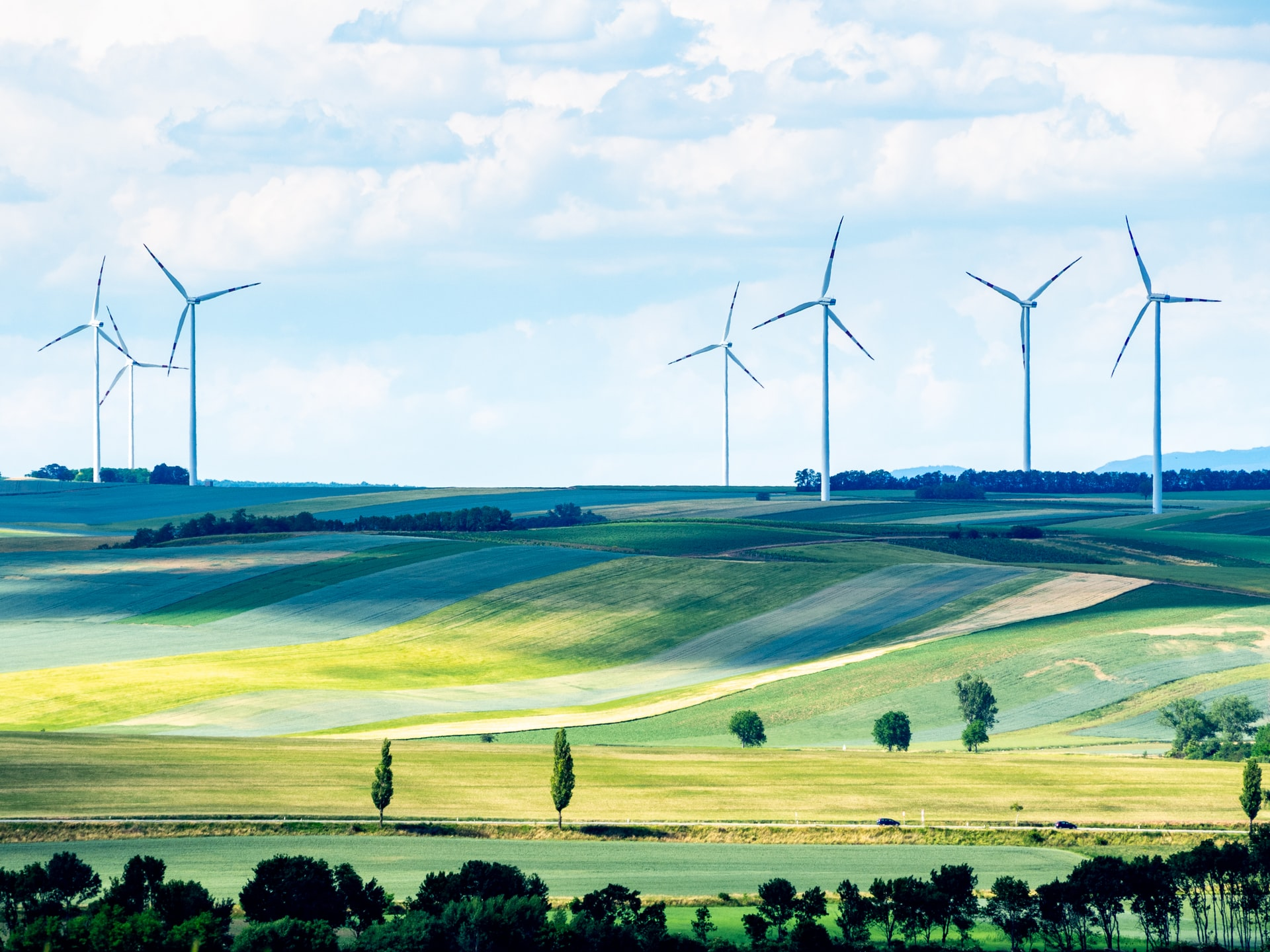# Wind Turbine Noise CalculatorThe Wind Turbine Noise Calculator is based upon the noise model presented in International Energy Agency: Expert Group Study on Recommended Practices for Wind Turbine Testing and Evaluation, 4. Acoustics Measurements of Noise Emission from Wind Turbines, 3. Edition 1994

This is a simplified version and uses hemi-spherical spreading over a reflective plane. The source sound power and absorption coefficient are both assumed to be broad band. An atmospheric absorption attenuation rate of 0.005dBm is used.

The following formula is used:

Lp = Lw – 10log10(2πr2) – ar

Where:

Lp is the calculated noise level (dB)
Lw is the sound power level of the wind turbine (dB)
r is the distance from source to receiver (m)
a is the absorption due to the atmosphere (dB/m)

In order to use the tool you will need to enter the sound power (dB) of the wind turbine and the distance (m) of the wind turbine from the receptor.

Additional wind turbines can be added, and the tool will calculate the overall cumulative sound pressure level (dB) of multiple wind turbines at the receptor location.